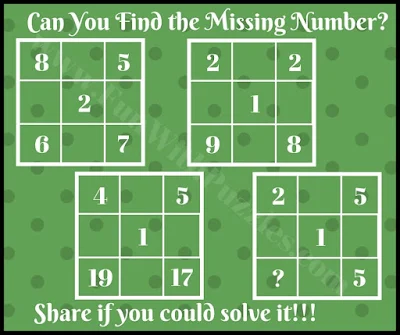This finding the missing number will test your brain. In this missing number puzzle, you are 5 numbers in the square. These numbers follow some logical pattern which relates these numbers. Can you decode this logical reasoning among the given numbers and then find the value of the missing number which will replace the question mark?Can you find the value of the missing number?
The answer to this "Finding the Missing Number", can be viewed by clicking on the answer button.